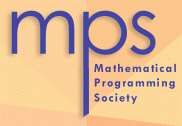2006 Fulkerson Prize Citation Manindra Agrawal, Neeraj Kayal and Nitin Saxena, "PRIMES is in P", Annals of Mathematics, Volume 160, issue 2, 2004, Pages 781--793. Testing whether an integer is a prime number is one of the most fundamental computational and mathematical problems. The existence of short certificates for both compositeness and primality was known since the 70's and suggested that primality testing might be in P. Yet, despite numerous efforts and a flurry of algorithms, it was not until 2002 that Agrawal, Kayal and Saxena devised the first deterministic polynomial-time algorithm for primality testing. Earlier algorithms had either assumed the generalized Riemann hypothesis, or been randomized or had been only subexponential. This is a stunning development. This result is a true masterpiece, combining algebraic and number theoretic results in a seemingly simple way. Mark Jerrum, Alistair Sinclair and Eric Vigoda, "A polynomial-time approximation algorithm for the permanent of a matrix with nonnegative entries", J. ACM, Volume 51, Issue 4, 2004, Pages 671--697. The permanent of a matrix has been studied for over two centuries, and is of particular importance to statistical physicists as it is central to the dimer and Ising models. For a 0-1 matrix, it represents the number of perfect matchings in the corresponding bipartite graph. Although polynomial-time computable for planar graphs, the computation of the permanent is #P-complete for general graphs as shown by Valiant in 1979. This opened the search for approximation schemes. In this paper, Jerrum, Sinclair and Vigoda give the first Fully Polynomial Randomized Approximation Scheme for computing the permanent of any 0-1 matrix or any nonnegative matrix. This is a remarkable result. Their algorithm is based on updating a Markov chain in a way that quickly converges to a rapidly mixing non-uniform Markov chain on perfect matchings and near-perfect matchings. Their work builds upon the earlier pioneering work of Jerrum and Sinclair who initiated the use of rapidly mixing Markov chains for combinatorial problems. Neil Robertson and Paul D. Seymour, "Graph Minors. XX. Wagner's conjecture", Journal of Combinatorial Theory, Series B, Volume 92, Issue 2 , 2004, Pages 325--357. Kuratowski's theorem says that a graph is planar if and only if it does not contain K_5 or K_{3,3} as a minor. Several other excluded minor characterizations are known, and Wagner conjectured that any minor-closed graph property can be characterized by a finite list of excluded minors. Restated, this says that for any infinite family of finite graphs, one of its members is a minor of another one. In a remarkable tour de force, Robertson and Seymour proved Wagner's conjecture, and this paper appeared as part 20 of their monumental work on the theory of graph minors. Their proof of the Graph Minor Theorem required the development of many graph theoretic concepts, such as linkages and tree-width. This is a spectacular achievement in graph theory with far reaching consequences. It shows, for example, that embeddability in any fixed surface can be characterized by a finite list of excluded minors, or that the disjoint paths problem can be solved in polynomial time for a fixed number of terminals.top of pageclose this window